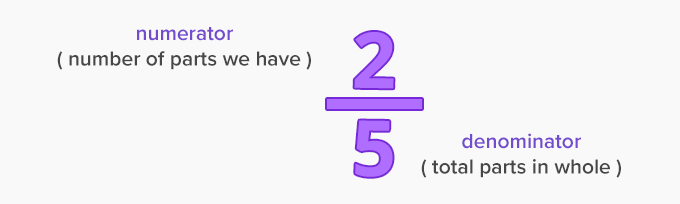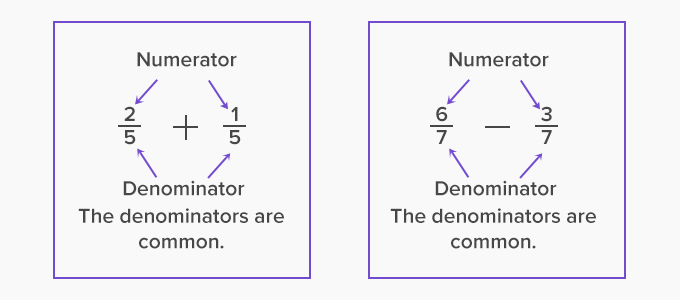# Common Denominator - Definition with Examples

The Complete K-5 Math Learning Program Built for Your Child

• 30 Million Kids

Loved by kids and parent worldwide

• 50,000 Schools

Trusted by teachers across schools

• Comprehensive Curriculum

Aligned to Common Core

## What is Common denominator?

A fraction has two parts – a numerator and a denominatorAny arithmetic operation such as addition or subtraction involving two or more fractions is possible if the denominators of both fractions are the same. This is known as the common denominator.

One can add or subtract fractions only when they have a common denominator. (see example below)A common denominator is a number with which both denominators share at least one factor other than 1.

## Fractions without a common denominator

We can obtain common denominators by multiplying both numerator (top) and denominator (bottom) by the same amount.

For example, consider the addition of two fractions that do not have the same denominator: